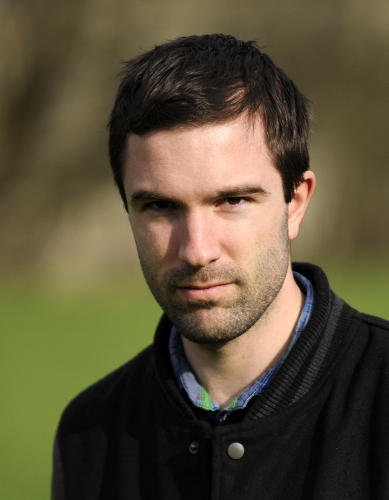I am a Research Assistant at Imperial College London working in the Centre for Mathematics of Precision Healthcare, under the supervision of Sophia Yaliraki and Mauricio Barahona. I was a PhD student at Imperial College London under the supervision of Darryl D. Holm. My PhD research lied at the interface of geometric mechanics, integrable systems and stochastic mechanics. Apart from these topic, I am now interested in dynamical systems on graphs and dimensionality reduction algorithms. Prior to Imperial College I did a Bachelor and Master in Physics at Ecole Polytechnique Fédérale de Lausanne, with a specialisation in Mathematics. For my Master thesis I also worked in the field of computational astrophysics with Lastro.

Coming talks:

Previous talks:

Posters:

# Videos

I put here videos illustrating some of my works.

## Videos of heavy top lattice

Below are the videos of the heavy top lattice with $\mathbb I= \mathrm{diag}(1,2,3)$ and $\mathbb J = \mathrm{diag}(1,2,3)$ (joint work with S. Takao).

## Videos of $Diff$-strands peakon anti-peakon collision

Video of Arnaudon, Holm, Ivanov 2017. Video: mov

## Videos of random attractors

Below are the videos of the random attractors on coadjoint orbits.

• Random attractor on the free rigid body. Video: mov
• Random attractor on the heavy top. Video: mov

## Videos of the CH-NLS equation

Below are a few videos of simulations of the CH-NLS equation. This equation was studied in arXiv:1507.02591 and corresponds to the figures of this paper. The CH-NLS equation reads $$im_t = u_{xx} + m(|u|-\alpha^2|u_x|),\qquad m=u-\alpha^2u_{xx}.$$

• Figure 2(b): Fission of an initial pulse into two solitons and radiations. Video: mov
• Figure 3(a): Collision of two solitary waves, similar to the NLS equation. Video: mov
• Figure 3(b): Collision of two solitary waves, with a narrow profile. Video: mov
• Figure 3(c): Collision of two solitary waves, with a higher speed. Video: mov